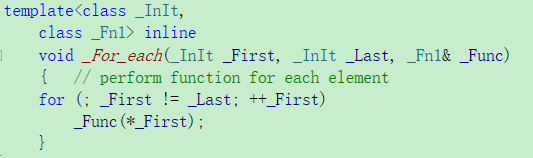## C++ 仿函数和适配器 转

o
osc_z1hvg4cu

#仿函数

``````bool greaterThan8(int n){
return n > 8;
}
int count = count_if(vec.begin(), vec.end(), greaterThan8);
``````

count_if第三个参数接收的是一个函数指针，并且这个函数指针只接收一个参数，返回值是bool。 count_if是将参数1和参数2之间的每一项都会作为参数去调用第三个参数，然后返回第三个参数每一次true的次数。

``````bool greaterThanN(int n, int x){
return n > x;
}
``````

``````int minValue = 8;
bool greaterThanN(int n){
return n > minValue ;
}
``````

2、没有可扩展性 　　如果我们每遇到一个类似的问题就新建一个全局变量，尤其是多人合作写代码时，很容易引起命名空间污染（namespace polution）的问题。

3、全局变量的问题 　　全局变量花销很大，一般是编程过程中极力避免的

``````\#define VALUE 8
bool greaterThanN(int n){
return n > VALUE;
}
``````

3.成员变量 我们既想要它既能想普通函数一样传入给定数量的参数，还能存储或者处理更多我们需要的有用信息。 如果存成成员变量，那成员函数调用不就是很正常的事了吗？

``````class functor {
public:
functor(int x) { value =x; }
void operator()(const int& x) { cout << x+value << endl;}
private:
int value;
};

functor fun(10);
fun(5); //15
``````

``````template<typename T>
class greater{
public:
greater(const T&x) { info = x; }
bool operator()(const T& x) { return info > x; }
private:
T info;
};
``````

``````greater<int> ge(20);
int count = count_if(vec.begin(), vec.end(), ge);
``````

``````int count = count_if(vec.begin(), vec.end(), greater<int>(20));
``````

1. 不能满足STL的抽象要求 参数，返回值如何设置
2. 无法和STL其他组件交互
``````template<typename _Kty, typename _Pr = less<_Kty>,...>
class set{...};

class Person{...};

std::set<Person, std::less<Person> > set1, set2; //operator<做排序行为
std::set<Person, std::greater<Person> >set3,set4;//operator>做排序行为

set1 = set2;  //正确，相同的型别
set1 = sete3; //错误，不同的型别！
``````

STL中也预先实现了一些仿函数，需要包头文件#include<functional>

``````#include <vector>
#include <iostream>
#include <algorithm>
#include <functional>
using namespace std;
int main()
{
std::vector<int> c{ 1, 5, 3, 4, 5, 6, 7 };
int x = 5;
int k=std::count_if(c.begin(), c.end(), [x](int n){return x == n; });
cout << k << endl;
return 0;
}
``````

#适配器 ###bind1st/bind2nd

``````vector<int> vec{0,0,0,10};
``````

``````std::vector<int>::iterator it = std::find_if(vec.begin(), vec.end(),
std::not_equal_to<int>(0));
``````

• `not_equal_to`结构没有一个参数的构造函数
• `not_equal_to``operator()`需要接受两个参数
``````template<class _Ty = void>
struct not_equal_to
{	// functor for operator!=
typedef _Ty first_argument_type;
typedef _Ty second_argument_type;
typedef bool result_type;

_CONST_FUN bool operator()(const _Ty& _Left, const _Ty& _Right) const
{	// apply operator!= to operands
return (_Left != _Right);
}
};
``````

``````typename std::not_equal_to<int>::first_argument_type nonZeroItem(0);
std::not_equal_to<int> f;
std::vector<int>::iterator iter = find_if(vec.begin(), vec.end(),
std::binder1st<std::not_equal_to<int> >(f, nonZeroItem));
``````

``````template<class _Fn2,
class _Ty> inline
binder1st<_Fn2> bind1st(const _Fn2& _Func, const _Ty& _Left)
{	// return a binder1st functor adapter
typename _Fn2::first_argument_type _Val(_Left);
return (binder1st<_Fn2>(_Func, _Val));
}
``````

``````	std::vector<int>::iterator iter = find_if(vec.begin(), vec.end(),
std::bind1st(std::not_equal_to<int>(), 0));
``````

`binder2nd``binder1st`类似。区别只在于`_Func`操作的是左值还是右值。 也就是说以下两句代码的同义的

``````std::vector<int>::iterator iter = find_if(vec.begin(), vec.end(),
std::bind1st(std::less<int>(), 0));  //std::bind2nd(std::greater<int>(), 0)

std::vector<int>::iterator iter = find_if(vec.begin(), vec.end(),
std::bind2nd(std::greater<int>(), 0));
``````

bind1st操作的是左值，找到第一个X，使满足0 < X bind2nd操作的是右值，找到第一个X，使满足X > 0

###mem_fun/mem_fun_ref 设计和上面类似，是用于适配对象的函数

``````	std::vector<Person*> vec;
vec.push_back(new Person("I",1));
vec.push_back(new Person("love", 2));
vec.push_back(new Person("u", 3));
vec.push_back(new Person("so much", 4));

std::for_each(vec.begin(), vec.end(), &Person::Print); //编译不通过
``````

``````1. fun(obj)  //fun是全局函数，非成员函数
2. obj.fun()  //obj非指针，fun是成员函数
3. obj->fun() //obj指针对象，fun是成员函数
``````

`for_each`会调用`_For_each` 只接收`fun(obj)`的调用``````std::for_each(vec.begin(), vec.end(), std::mem_fun(&Person::Print));
``````

`mem_fun_ref`类似，但是用于容器中存放的不是指针的情况

``````方法一
for (vector<Person*>::iterator it = vec.begin(); it != vec.end(); ++it)
delete (*it);
vec.clear();

struct DeleteItem {
template<typename _Ty>
void operator()(const _Ty p) const
{
delete (p);
}
};
std::for_each(vec.begin(), vec.end(), DeleteItem());
vec.clear();
``````

#####注意 1、有继承的情况下，容器存放指针而不是对象

• 对象拷贝比指针拷贝对象大得多
• 父对象的容器存放子对象时，数据会Slice

2、使用指针记得释放内存 3、尽量用算法代替手写循环 代码更优雅，据说速度更快，但是还没测试过

o

### osc_z1hvg4cu

C++ STL概论

STL六大组件介绍 STL(Standard Template Library) 是一个组件集合，共包含以下六大组件，彼此可以组合套用： 1、容器（containers）：包含各种数据结构，如vector、list、deque、set、map，用...

Spring_24
04/08
0
0

romalin99
2015/04/02
3
0
C++模板技术与STL实战开发-夏曹俊-专题视频课程

C++模板技术与STL实战开发—1006人已学习 课程介绍 泛型编程（Generic Programming）是一种全新的程序设计思想，它和STL是现代C++的精髓。可以说，不会STL很难称得上掌握了现代C++编程。STL...

06/30
0
0
C++ STL介绍——简介

@[TOC]目录 1、什么是STL STL，即标准模板库，是一个具有工业强度的，高效的C++ 程序库。它被容纳于C++ 标准程序库中，是 标准中最新的也是极具革命性的一部分。该库包含了诸多在计算机科学领...

osc_arhujamz
2019/07/26
1
0
STL仿函数与函数对象浅析

05/18
0
0

R中“ =”和“ <-”赋值运算符有什么区别？

fyin1314
25分钟前
12
0
JavaScript中的静态变量 - Static variables in JavaScript

14
0

javail

15
0

7
0

10
0